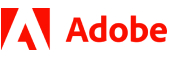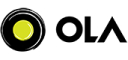New update is available. Click here to update.
Topics

# House Robber II

Moderate0/80
Average time to solve is 15m+14 more companies

## Problem statement

Mr. X is a professional robber planning to rob houses along a street. Each house has a certain amount of money hidden. All houses along this street are arranged in a circle. That means the first house is the neighbor of the last one. Meanwhile, adjacent houses have a security system connected, and it will automatically contact the police if two adjacent houses were broken into on the same night.

You are given an array/list of non-negative integers 'ARR' representing the amount of money of each house. Your task is to return the maximum amount of money Mr. X can rob tonight without alerting the police.

Note:
``````It is possible for Mr. X to rob the same amount of money by looting two different sets of houses. Just print the maximum possible robbed amount, irrespective of sets of houses robbed.
``````
For example:
``````(i) Given the input array arr[] = {2, 3, 2} the output will be 3 because Mr X cannot rob house 1 (money = 2) and then rob house 3 (money = 2), because they are adjacent houses. So, he’ll rob only house 2 (money = 3)

(ii) Given the input array arr[] = {1, 2, 3, 1} the output will be 4 because Mr X rob house 1 (money = 1) and then rob house 3 (money = 3).

(iii) Given the input array arr[] = {0} the output will be 0 because Mr. X has got nothing to rob.
``````
Detailed explanation ( Input/output format, Notes, Images )
Constraints:
``````1 <= T <= 10
1 <= N <= 5 x 10 ^ 3
1 <= ARR[i] <= 10 ^ 9

Time limit: 1 sec.
``````
Sample Input 1:
``````3
1
0
3
2 3 2
4
1 3 2 1
``````
Sample Output 1:
``````0
3
4
``````
Explanation of Input 1:
``````(i) Mr. X has only one house to rob, but with no money.

(ii) Mr. X cannot rob house 1 (money = 2) and then rob house 3 (money = 2), because they are adjacent houses (remember, it’s a circular street). So, he’ll rob only house 2 (money = 3) with a maximum value

(iii) Mr. X will get maximum value when he robs house 2 (money = 3) and then robs house 4 (money = 1) i.e. 4 units of money.
``````
Sample Input 2:
``````3
5
1 5 1 2 6
3
2 3 5
4
1 3 2 0
``````
Sample Output 2:
``````11
5
3
``````Console